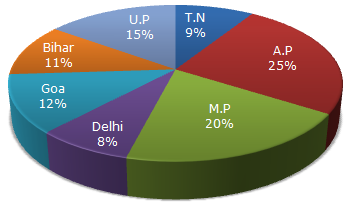# Data Interpretation - Pie Charts - Discussion

Study the following graph and the table and answer the questions given below.

Data of different states regarding population of states in the year 1998Total population of the given States = 3276000.

 States Sex and Literacy wise Population Ratio Sex Literacy M F Literate Illiterate A.P 5 3 2 7 M.P 3 1 1 4 Delhi 2 3 2 1 Goa 3 5 3 2 Bihar 3 4 4 1 U.P. 3 2 7 2 T.N. 3 4 9 4

5.

If in the year 1998, there was an increase of 10% in the population of U.P. and 12% in the population of M.P. compared to the previous year, then what was the ratio of populations of U.P. and M.P. in 1997?

 [A]. 42 : 55 [B]. 48 : 55 [C]. 7 : 11 [D]. 4 : 5

Explanation:

Let x be the population of U.P. in 1997. Then,

 Population of U.P. in 1998 = 110% of x = 110 x x. 100

Also, let y be the population of M.P. in 1997. Then,

 Population of M.P. in 1998 = 112% of y = 112 x y. 100

Ratio of populations of U.P. and M.P. in 1998 =110 x x100
= 110x .112 x y100
112y

 From the pie-chart, this ratio is 15 . 20110x = 15x = 15 x 112 = 42 . 112y 20 y 20 110 55

Thus, ratio of populations of U.P. and M.P. in 1997 = x : y = 42 : 55.

 Satya said: (Jul 29, 2015) Can any one explain it?

 King said: (Nov 14, 2015) Why not this? (15/100)*100/110 : (20/100)*100/120. = (3/20)*(10/11) : (1/5)*(5/6). = 3/11 : 1/6 = 18:22. = 9:11.

 Yara said: (May 29, 2016) Could it be explained in any other way?

 Monil said: (Jun 30, 2017) Can it be written in these way also or not? ( 90% of 15% of total ) ÷ ( 88% of 20% of total). = ( 90 * 15 * N ) ÷ ( 88 * 20 * N ). = 135 ÷ 176.

 Satish said: (Aug 28, 2017) {(15*100)÷110}:{(20*100)÷112}. 42 : 55.

 Ank said: (Jun 26, 2018) Can it be written in these way also or not? ( 90% of 15% of total ) ÷ ( 88% of 20% of total). = ( 90*15*N ) ÷ ( 88*20*N). = 135 ÷ 176.

 Haise said: (Jul 17, 2019) How come 110 & 112? Can anyone explain?